## General Question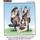# Can one explain this math error? (Not homework)

Asked by RedDeerGuy1 (20529) 1 month ago

1 Kilogram squared vs. 1000 Grams squared ?

Are they the same?
What other math inconsistencies are there?

Does this cause a problem in measurements?

Observing members: 0Composing members: 0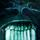They are not the same because 1000 is bigger than 1.

The number 1 squared = 1*1 = 1

The number 1000 squared = 1000*1000= 1000000

Even though 1Kg = 1000g once you have changed the units you have moved the 000s from the units in to the actual number. Humans think of them as interchangeable but maths just works on what is there.

Lightlyseared (33366)“Great Answer” (1) Flag as…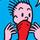I’m with @Lightlyseared on this one. You have numbers and you have units. Math looks at both. If you were trying to compare two different units, you’d have to put them into common units so you were talking apples to apples (or grams to grams). But the comparison you are using is trying to do just the numbers without the units. 1 squared is 1. 1000 squared is 1,000,000. So the numbers were not equal to begin with…1 does not equal 1000. So you can’t square just the numbers and ignore the units if you are assuming the two sides of the equation were equal to begin with.

seawulf575 (14136)“Great Answer” (1) Flag as…@seawulf575 I am still wondering….

RedDeerGuy1 (20529)“Great Answer” (0) Flag as…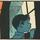The error isn’t in the math. The error is in the concept. You cannot “square” a kilogram. There is no measure that is one kilogram by one kilogram.

You can “square” a kilometer, because then you are talking linear measures. And a square kilometer is the same size as 1,000 meters squared.

zenvelo (37560)“Great Answer” (5) Flag as…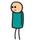Yes, they are the same. See dimensional analysis.

bob_ (21437)“Great Answer” (2) Flag as…@zenvelo has it right. There is no such thing as a square gram. A square kilometer is 1000 meters by 1000 meters so a square kilometer equals 1000×1000=1,000,000 square meters.

One ml of water weighs 1 gram, so a liter of water weighs 1000 grams = 1 kg. There are 1000 liters in a cubic meter, so a cubic meter of water weighs 1,000 kg. A cubic kilometer is 1000×1000x1000 = a billion cubic meters, so a cubic kilometer of water would weigh a billion times 1000 kg = 1 trillion kg

LostInParadise (29757)“Great Answer” (4) Flag as…or